# Derive the expression for electric field intensity due to a uniformly charged thin spherical shell

Derive the expression for electric field intensity due to a uniformly charged thin spherical shell.

Let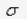the uniform surface charge density of a thin spherical shell of radius R .Let us find out electric field intensity at a point P outside or inside the shell.

1. Field outside the shell-
Let us find out electric field intensity at a point P outside the spherical shell, such that OP=r. Here we take Gaussian surface as a sphere of radius r. the electric field intensity,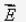is same at every point of Gaussian surface, directed radially outwards (as is unit vector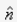, so that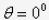)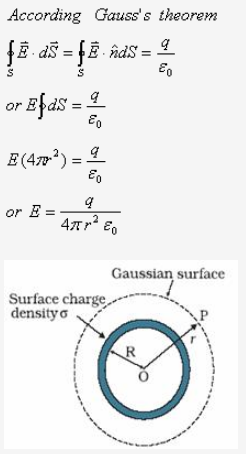Hence it is clear that electric intensity at any point outside the spherical shell is such, as if the entire charge is concentrated at the centre of the shell.

2. Field at the surface of the shell-
For this we have r = R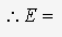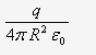Ifis the charge density on the shell, then3. Field inside the shell -
If the point P lies inside the spherical shell, then Gaussian surface is a surface of sphere of radius r
As there is no charge inside the spherical shell, Gaussian surface encloses no charge.That is q=0
Hence the field inside the spherical shell is always zero.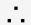E = 0
Hence the field inside the spherical shell is always zero.